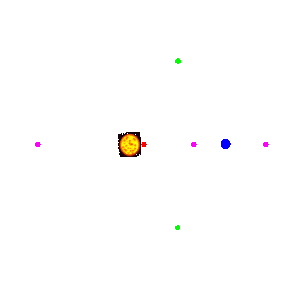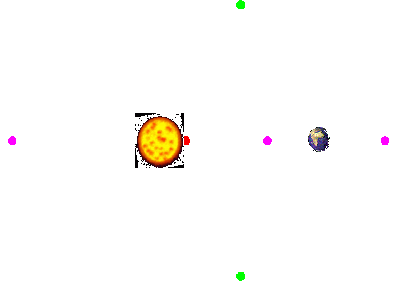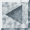Libration Points

There exist five fixed points in the CRTBP. Also known as Lagrange, or Libration points, these points represent points in space where an unmoving particle would remain for all time. These fixed points are all points where all six phase variables time derivatives are zero. Two of these points, known as L4 and L5 form equilateral triangles with the primary masses. The other three libration points (L1, L2, L3) are colinear with the primary masses, and are found by solving quintic polynomials dictated by the equations of motion.Key

Sun

Earth

Center of mass

Colinear libration points (L1, L2, L3)

Libration points (L4, L5)

These libration points co-rotate with the system (i.e. they do note change postion with respect to the primary bodies because the bodies are in motion. This animation shows the co-rotating system with its 5 libration points.This figure shows the labeled libration points in a non-rotating frame of reference.Circular Restricted Three Body menu